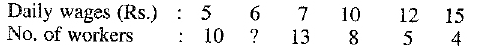Maths-
General
Easy

Question

# A frequency distributionhas arithmetic mean 7.85, then missing term is

## 10 5    10 05    15 5    15Hint:

## The correct answer is: 15

### The formula of arithmetic mean isHere xi is the quantity whose frequency we measure.f is the frequency of that quantity.N is sum of all the frequencies. Here it means total number of workers.N = 10 + x + 13 + 8 + 5 + 4= 40 + xWe are given m = 7.85Now, we will substitute all the values and find the answer.So, the missing term is 15.

For such questions, we need the the formula for arithmetic mean.#### With Turito Foundation.#### Get an Expert Advice From Turito.